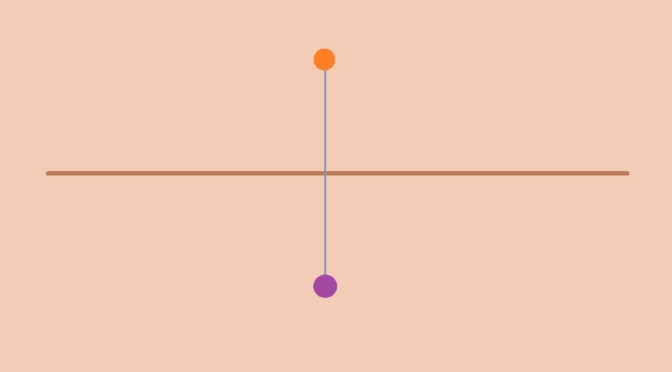# The line with two origins

Let’s introduce and describe some properties of the line with two origins.

Let $$X$$ be the union of the set $$\mathbb R \setminus \{0\}$$ and the two-point set $$\{p,q\}$$. The line with two origins is the set $$X$$ topologized by taking as base the collection $$\mathcal B$$ of all open intervals in $$\mathbb R$$ that do not contain $$0$$, along with all sets of the form $$(-a,0) \cup \{p\} \cup (0,a)$$ and all sets of the form $$(-a,0) \cup \{q\} \cup (0,a)$$, for $$a > 0$$.

### $$\mathcal B$$ is a base for a topology $$\mathcal T$$ of $$X$$

Indeed, one can verify that the elements of $$\mathcal B$$ cover $$X$$ as $X = \left( \bigcup_{a > 0} (-a,0) \cup \{p\} \cup (0,a) \right) \cup \left( \bigcup_{a > 0} (-a,0) \cup \{q\} \cup (0,a) \right)$ and that the intersection of two elements of $$\mathcal B$$ is the union of elements of $$\mathcal B$$ (verification left to the reader).

### Each of the spaces $$X \setminus \{p\}$$ and $$X \setminus \{q\}$$ is homeomorphic to $$\mathbb R$$

Let’s prove it for $$X \setminus \{p\}$$. The map $\begin{array}{l|rcll} f : & X \setminus \{p\} & \longrightarrow & \mathbb R \\ & x & \longmapsto & x & \text{for } x \neq q\\ & q & \longmapsto & 0 \end{array}$ is a bijection. $$f$$ is continuous as the inverse image of an open interval $$I$$ of $$\mathbb R$$ is an open subset of $$X$$. For example taking $$I=(-b,c)$$ with $$0 < b < c$$, we have \begin{align*} f^{-1}[I] &= (-b,0) \cup \{q\} \cup (0,c)\\ &= \left( (-b,0) \cup \{q\} \cup (0,b) \right) \cup (b/2,c) \end{align*} One can also prove that $$f^{-1}$$ is continuous.

### $$X$$ is not Hausdorff

If $$X$$ was a Hausdorff space, there would exist disjoint open subsets $$U, V$$ containing respectively $$p$$ and $$q$$. Hence there would exist positive reals $$a,b$$ such that $$(-a,0) \cup \{p\} \cup (0,a)$$ and $$(-b,0) \cup \{q\} \cup (0,b)$$ are disjoint. Which is absurd.

### $$X$$ satisfies all the conditions for a manifold except for the Hausdorff condition

Indeed, we have proven that $$X$$ is a topological space and that each point is locally homeomorphic to $$\mathbb R$$. One can also verify that $$X$$ is second countable as all open intervals in $$\mathbb R$$ having rational endpoints that do not contain $$0$$, along with all sets of the form $$(-a,0) \cup \{p\} \cup (0,a)$$ and all sets of the form $$(-a,0) \cup \{q\} \cup (0,a)$$, for $$a > 0$$ rational, form a base of the topology of $$X$$.